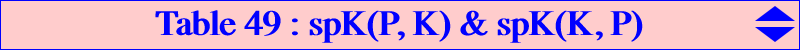The cubics spK(P, Q) are defined and studied in CL055. spK(P, Q) is a K0 (a cubic without term in xyz) for any P (resp. Q) if and only if Q (resp. P) is the Lemoine point K = X(6). This gives two nets of cubics which are studied below.The net of cubics spK(P, K) Any cubic spK(P, K) is a circum-cubic passing through the (four) foci of the inconic (K) with center K sometimes called "ellipse K" (Brocard & Lemoyne, courbes géométriques, tome 3, p. 227). It is actually an ellipse when ABC is acute angled. spK(P, K) also contains the reflection P' of P about K, the isogonal conjugate P* of P, the infinite points of pK(K, P) and those of pK(K, P') on the circum-circle. Note that spK(P, K) and spK(P', K) are two isogonal conjugate cubics which coincide when P = K (giving the Grebe cubic K102) or when P lies on the line at infinity (see below). This net contains several remarkable cubics : • one stelloid spK(O, K) = K708. • a pencil of isogonal focal cubics when P lies on the line at infinity. These are nK0s with root on the orthic axis and singular focus on the circum-circle. • another pencil of nK0s when P lies on the circum-conic with center K, perspector O. Their poles lie on cK(#X6, X25) and their roots lie on the circum-circle. • a family of pKs when P lies on the central cubic K707 = pK(X3, X193). In this case, their poles lie on K171 = pK(X32, X25), their pivots lie on K233 = pK(X25, X4), their isopivots lie on K168 = pK(X3, X2). • a family of K+s when P lies on a cubic passing through X(1), X(2), X(3), X(194), the excenters and the infinite points of the Grebe cubic K102. For example, spK(G, K) is K314 which is a central cubic. Here is a selection of these cubics spK(P, K).P spK(P, K) type centers and/or remark X(524) K018 nK0 focal cubic X(511) K019 nK0 focal cubic X(518) K040 cK0 strophoid X(6) K102 pK X(3564) K164 nK0 focal cubic X(193) K168 pK X(542) K188 nK0 focal cubic X(523) K189 nK0 focal cubic X(525) K190 nK0 focal cubic X(69) K233 pK X(2) K314 K++ central cubic X(3) K708 K+ stelloid X(1) -- K+ X(1) X(110) -- nK0 X(523), X(895), X(2574), X(2575) X(194) -- K+ X(194), X(3224) X(698) -- nK0 X(194), X(698), X(699), X(3224) / focal cubic X(895) -- nK0 X(110), X(468), X(1113), X(1114)The net of cubics spK(K, P) Recall that spK(K, P) is a circum-cubic K0 passing through G, the reflection P' of K about P, the infinite points of K102, the points of pK(X6, P') on the circumcircle and the foci of the inconic with center P. It follows that spK(K, P) cannot be circular nor equilateral. Furthermore, any spK(K, P) with P at infinity and not on K102 must split into the line at infinity and a circum-conic. • spK(K, P) is a nK0 when P lies on the bicevian conic C(G, K) which is the complement of the circum-conic with center X(141), perspector X(39). The pole lies on this latter conic and the root on cK(#X2, X76). • spK(K, P) is a pK when P lies on the complement of the central cubic K140 = pK(X39, X69). In this case, its pivot lies on K141 = pK(X2, X76) and its isopivot lies on pK(X39, X2). For instance, spK(K, K) is K102 and spK(K, X141) is K141. • spK(K, P) is a K+ when P lies on the line passing through G and the midpoint of X(6), X(39). In this case, the intersection of the asymptotes lies on the line X(2), X(39), X(76),X(194),X(274), etc. When P is X(2), the cubic is the central cubic K287 and when P is the midpoint of X(6)X(39), we obtain another central cubic with center X(39).